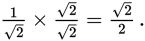# SAT Math Multiple Choice Question 264: Answer and Explanation

### Test Information

Question: 264

9. Which of the following expressions has the same value as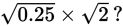• A.• B.• C.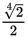• D.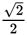Explanation:

D

Difficulty: Medium

Category: Passport to Advanced Math / Exponents

Strategic Advice: Use the rules for radicals to simplify the product. Don't actually try to find the value of each answer choice.

Getting to the Answer: When two radical expressions with the same degree root are multiplied, you can multiply the numbers under the radicals, leaving the product inside. The root stays the same. Writing 0.25 as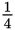may make finding the product easier: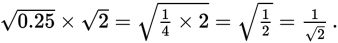It's not proper to leave a radical in the denominator (and this is not one of the answer choices), so rewrite the expression by multiplying the top and bottom by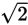to get